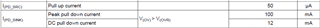If you have a related question, please click the "Ask a related question" button in the top right corner. The newly created question will be automatically linked to this question.

# LM74722-Q1: how to calculate turn on time?

Part Number: LM74722-Q1
Other Parts Discussed in Thread: LM74700-Q1

Hi Expert,

Our customer plan to use LM74722-Q1 to drive 6pcs IAUC120N06S5N017. How to calculate the turn on time? May I know the source current of GATE pin?

And why Zener diode  is only needed for Q2? Thanks.

BR,

Elec Cheng

• Hi Elec,

The Gate drive strength of LM74722-Q1 can be estimated using the tGATE_ON(DLY) parameter as shown below.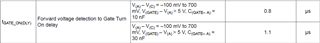tGATE_ON(DLY) can be considered as sum of tDELAY + tRISE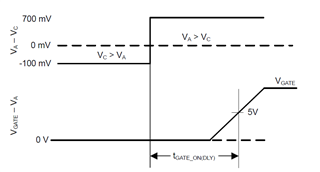From the table above the total Gate Turn ON Delay for gate capacitance of 10nF is 0.8us while for 30nF cap it is 1.1us. From this data, the  tDELAY can be estimated to be around 0.65us. Using this data, you can calculate the tGATE_ON(DLY) for a given CISS of the total No. of FETs.

• Hi Praveen，

sorry that I still don't get it how to calculate the GATE pin's source current and the turn on delay. Could you please share more detail?

What does Icap mean? Also inside the chip, how does GATE pin voltage and PD pin voltage come from? Why do they output different voltage?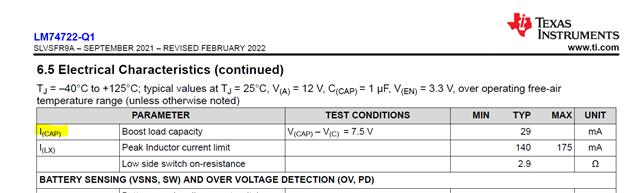And I don't see PD pin's sink current and source current. Could you please share?

BTW, I see from LM74700-Q1 datasheet and it shows the parameter as below. Why don't we have a clear parameter for LM74722-Q1?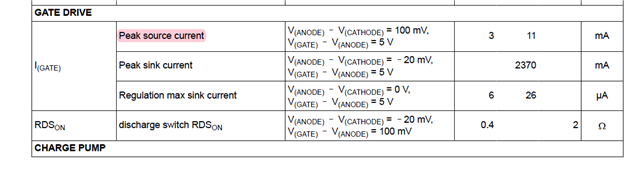Thanks for help!

BR,

Elec Cheng

•  Hi Elec,

1) Continuing the explanation from my previous post,

tGATE_ON(DLY) = tDELAY + tRISE

tGATE_ON(DLY) = 0.65us + tRISE

For 10nF, tGATE_ON(DLY) = 0.8us . this means the tRISE = 0.15us

From Igate = Ciss x (dv/dt) , where dt = tRISE , dV = 5V

⇒ Igate = 10nF x 5V / 0.15us = 333.3 mA

Say for example you have FETs in parallel with total Ciss = 40nF, then

tGATE_ON(DLY) = 0.65us + [Ciss x (dv) / Igate] = 0.65us + [40nF x 5 / 333.3mA] = 0.65us + 0.6us = 1.25us

LM74722-Q1 device has a very fast gate drive capability because of which the tDELAY parameter is a significant contributor to the total gate turn ON delay.

2) I(CAP) is the boost loading capacity. This is the max current which can be supported by the integrated boost converter. This parameter is important to consider only during estimation of the controllers AC superimposed signal rectification capability. For more understanding please refer to Ideal Diode Controllers for Active Rectification of AC Voltage Ripple Application Note.

3) The integrated boost converter generates a voltage greater than input voltage. This boost converter output voltage is used to drive PD and GATE pins. The voltages for PD and GATE are different because the drivers are different. PD is driven w.r.t GND but GATE is driven w.r.t A pin internally.

4) The PD pin Source and Sink current capability is specified in the datasheet